# Bukhvalov theorem

(diff) ← Older revision | Latest revision (diff) | Newer revision → (diff)

on kernel operators

The theory of kernel operators (cf. also Kernel of an integral operator) was essentially influenced by N. Dunford and B.J. Pettis [a2] around 1940. Other important results were obtained at about the same time by L.V. Kantorovich and B.Z. Vulikh. Among other results, it was shown that every bounded linear operator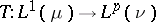,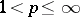, is a kernel operator in the sense that there exists a measurable functionsuch thatfor almost alland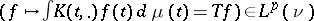.

This result is known as Dunford's theorem. In the decades following these first results, kernel operators were intensively studied. While the first results were mainly concerned with a single kernel operator or were in the spirit of Dunford's theorem, the investigation of the structure of the space of all kernel operators in the space of all regular operators began in the 1960s, following the study of Banach function spaces (cf. also Banach function space), or even ideal spaces in the space of measurable functions.

Under very general assumptions it was shown that the kernel operators ofform a band in the space of all regular operators, where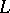andare ideals in the space of measurable functionsor, respectively. At that time many properties of kernel operators were known. It was A.V. Bukhvalov who gave [a1] a simple characterization of kernel operators, as follows.

Let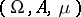,be measure spaces (cf. also Measure space), let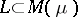andbe ideals such that the support of the Köthe dualis all of. Then for every linear operatorthe following conditions are equivalent:

i)is a kernel operator.

ii) If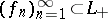is an order-bounded sequence which is star convergent, then the sequenceis convergent almost everywhere. Here, a sequence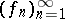said to be star convergent to someif every subsequence of the sequencecontains a subsequencesuch that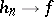almost everywhere as. Consequently,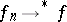, as, if and only if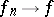in the measureon every subset of finite measure.

While the proof of i)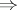ii) is a simple consequence of the Lebesgue convergence theorem (cf. also Lebesgue theorem), the proof of ii)i) requires many results concerning the structure of the space of kernel operators in the space of all regular operators. A simplified version of the proof is due to A.R. Schep [a4].

Bukhvalov's theorem is a powerful tool in the study of operators between Banach function spaces. In particular, his characterization of kernel operators leads to simple proofs of many classical results, such as Dunford's theorem and generalizations of it. For more information, see [a3], Sect. 3.3, or [a5].

How to Cite This Entry:
Bukhvalov theorem. Encyclopedia of Mathematics. URL: http://encyclopediaofmath.org/index.php?title=Bukhvalov_theorem&oldid=17381
This article was adapted from an original article by Peter Meyer-Nieberg (originator), which appeared in Encyclopedia of Mathematics - ISBN 1402006098. See original article